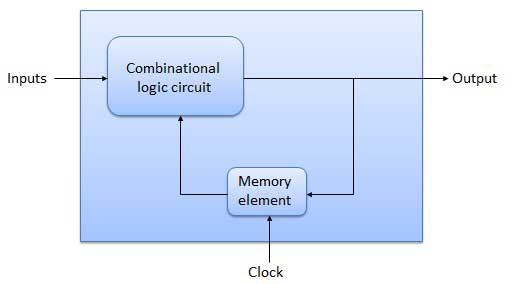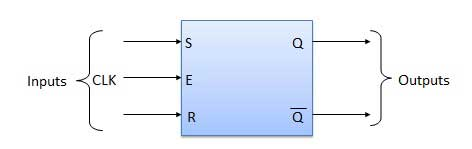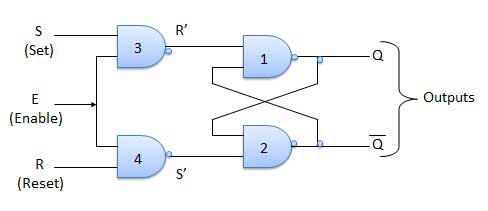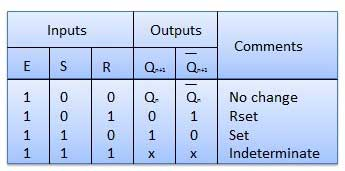### Sequential Circuits

• Most digital logic gates and digital logic systems use “Positive logic”, in which a logic level “0” or “LOW” is represented by a zero voltage, 0v or ground and a logic level “1” or “HIGH” is represented by a higher voltage such as +5 volts, with the switching from one voltage level to the other, from either a logic level “0” to a “1” or a “1” to a “0” being made as quickly as possible to prevent any faulty operation of the logic circuit.
• There also exists a complementary “Negative Logic” system in which the values and the rules of a logic “0” and a logic “1” are reversed but in this tutorial section about digital logic gates we shall only refer to the positive logic convention as it is the most commonly used.

• In standard TTL (transistor-transistor logic) IC’s there is a pre-defined voltage range for the input and output voltage levels which define exactly what is a logic “1” level and what is a logic “0” level and these are shown below

Block Diagram:Flip Flop:

• A flip flop is a sequential circuit that samples its inputs and changes its outputs just once in a while, rather than continually. Instead of being level triggered like latches, flip flops are known to be edge sensitive or edge triggered.

S-R Flip Flop:

• It's essentially an S-R latch with NAND gates and an enable input. Level activated SR-FF is another name for it. If and only if the enable input (E) is made active, the circuit in output will occur. In summary, this circuit will function as an S-R latch if E = 1, while the output will remain unchanged if E = 0.

Block Diagram:Circuit Diagram:Truth Table:S.N Condition Operation 1. S = R = 0 : No change If S = R = 0 then output of NAND gates 3 and 4 are forced to become 1.   Hence R' and S' both will be equal to 1. Since S' and R' are the input of the basic S-R latch using NAND gates, there will be no change in the state of outputs. 2. S = 0, R = 1, E = 1 Since S = 0, output of NAND-3 i.e. R' = 1 and E = 1 the output of NAND-4 i.e. S' = 0.   Hence Qn+1 = 0 and Qn+1 bar = 1. This is reset condition. 3. S = 1, R = 0, E = 1 Output of NAND-3 i.e. R' = 0 and output of NAND-4 i.e. S' = 1.   Hence output of S-R NAND latch is Qn+1 = 1 and Qn+1 bar = 0. This is the reset condition. 4. S = 1, R = 1, E = 1 As S = 1, R = 1 and E = 1, the output of NAND gates 3 and 4 both are 0 i.e. S' = R' = 0.   Hence the Race condition will occur in the basic NAND latch.